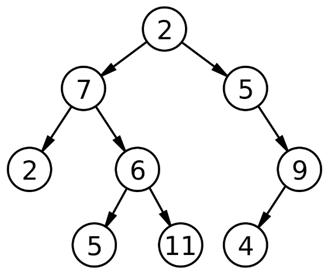# Level Order Traversal on a Binary Tree | Data Structure

In this article, we are going to learn Level order traversal on a binary tree: Inorder, Preorder and Postorder Traversal.
Submitted by Radib Kar, on September 29, 2018

For traversal on any binary tree, we mainly use three types of traversal. Those are:

1. Inorder traversal
2. Preorder Traversal
3. Postorder Traversal

But there is another kind of traversal technique, quite similar to BFS of the graph, known as "Level order traversal".

The level order traversal means traversing left to right level-wise. Level order traversal of the following example turns to be: 2, 7, 5, 2, 6, 9, 5, 11, 4.Image source: Wikipedia

The level order traversal is defined as follows:

• Visit the root
• While traversing level l, keep all elements at level l+1 in queue
• Go to next level and visit all the nodes at that level
• Repeat until all levels are completed

Pseudocode:

```struct BT{              // tree node type
int data;           //value
struct BT *left;    //pointer to left child
struct BT *right;   //pointer to right child
};

void levelorder (struct BT *root){ // root of the tree
struct BT *temp;                // BT refers to node of tree (datatype for the node);
struct Queue *q=Creat_queue();  //creating a queue

if(!root)   //root is null
return;

EnQueue(q,root); // Enqueue the root in the queue

while(!emptyQueue(q)){
temp=DeQueue(q);  //Dequeue
print(temp->data); //print the data
if(temp->left)
EnQueue(q,temp->left); // if left child exists EnQueue
if(temp->right)
EnQueue(q,temp->right); // if right child exists EnQueue
}
DeleteQueue(q);
}
```

## C++ implementation of level order traversal

```#include <bits/stdc++.h>
using namespace std;

class tree{    // tree node is defined
public:
int data;
tree *left;
tree *right;
};

void levelorder( tree *root){
queue<tree*> q;  // using stl
tree* temp;
q.push(root);
while(!q.empty()){
temp=q.front();
q.pop();
cout<<temp->data<<" ";  //process node
if(temp->left)
q.push(temp->left); //EnQueue
if(temp->right)
q.push(temp->right); //EnQueue
}
}
tree* newnode(int data)  // creating new node
{
tree* node = (tree*)malloc(sizeof(tree));
node->data = data;
node->left = NULL;
node->right = NULL;

return(node);
}

int main()
{
//**same tree is builted as shown in example**
tree *root=newnode(2);
root->left= newnode(7);
root->right= newnode(5);
root->right->right=newnode(9);
root->right->right->left=newnode(4);
root->left->left=newnode(2);
root->left->right=newnode(6);
root->left->right->left=newnode(5);
root->left->right->right=newnode(11);

cout<<"Level Order traversal of binary tree is :"<<endl;
levelorder(root);

return 0;
}
```

Output

```Level Order traversal of binary tree is :
2 7 5 2 6 9 5 11 4
```